Sample Midterm Exam 2 Solutions

Question 1 (10). Find the derivative of the following functions:
a.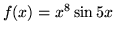Solution: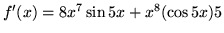by the product rule and the chain rule.

b.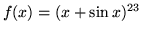Solution: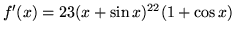by the chain rule.

c.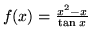Solution: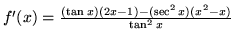by the quotient rule.

d.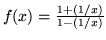Solution:
First simplify: this gives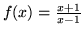Now it is easy to use the quotient rule: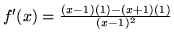by the quotient rule. This can be simplfied to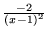e.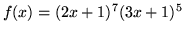Solution: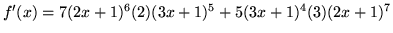by the product and chain rules.

Question 2 (15). a. State the definition of the derivative of a function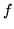at a point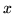.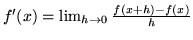, if this limit exists.

b. Use the definition of the derivative to compute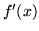for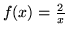.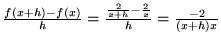Taking the limit of this as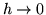, we obtain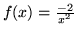.

Question 3 (10). Find all the vertical and horizontal asymptotes of the graph of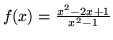We first notice that there is a simplification,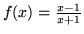Then we see that there is a vertical asymptote at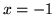. As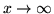, we have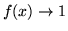, and similarly for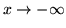. So there is one horizontal asymptote,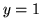, for bothand.

Question 4 (20). For each of the following, either find the limit or state that "no limit exists" and briefly explain why. Show work used to get your answer.

a.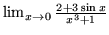Answer: 2. The limit can be obtained by plugging in, since this does not lead to division by zero or other problems, and the numerator and denominator are continuous.

b.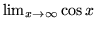.

Does not exist, since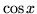oscillates between -1 and 1 and does not approach a single value.

c.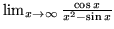.
Answer: 0, since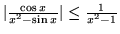forlarge, and this approaches zero as.

d.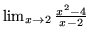.

Answer: 4, since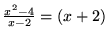when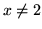, and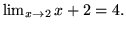Question 5 (10). For the function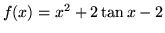a. Find the equation of the tangent line to the graph of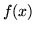at the point (0,-2).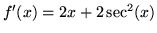and at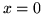this has value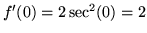. So the line has slope 2 and goes through the point (0,-2). Using the point-sl ope formula gives the equation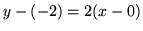or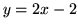.

b. Show that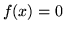at some point.
We know that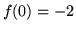and. The functionis continuous on the interval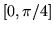, so by the Intermediate Value Theorem, there is a point in this interval whereQuestion 6 (10) a. State the precise definition of what is meant by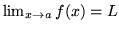.
Given an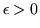there is a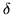such that whenever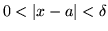then it is true that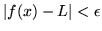.

Use the precise definition of the limit to prove that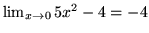.
To ensure that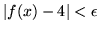, or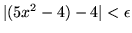, whatcan we allow? Simplifying gives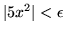, or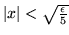. So we pick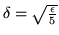and we satisfy the condition for the limit to equal 4.

Question 7 (5) Give an example of a functionwhich is continuous at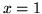but not differentiable at.

The function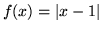is an example.

Question 8 (5) Supposeand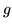are functions and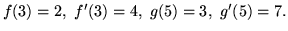Where can you calculate the derivative of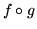? What is it equal to?
At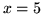, the chain rule tells us that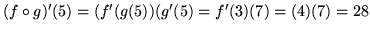Question 9 (5) Let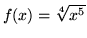. Find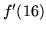.
By the chain rule,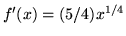and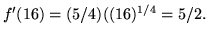Question 10 (10). Find an anti-derivative of the following functions:
a.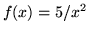.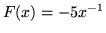.

b.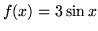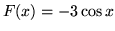.

c.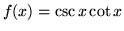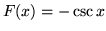.